# Density surface model for hare point transect survey

Point transects for hares analysed with model-based inference to study area.

Guillaume Souchay https://scholar.google.com/citations?user=mFKm9kAAAAAJ&hl=en (French National Hunting and Wildlife Agency)https://ofb.gouv.fr/
2020-09-08

In France, the French National Agency for Hunting and Wildlife has recently created the Hare network (Mauvy, Souchay, Arnauduc, & Guitton, 2017), a network of voluntary sites where hares and foxes are sampled every year in February using point transect sampling (Peroux, Mauvy, Lartiges, Bray, & Marboutin, 1997).

As an illustration, we used the sampling data from Rouillacais for hare only, in western part of France. At this site, ~ 50 points were sampled during 3 successive nights in February of 2015 and 2016, distance from observers to hares and foxes were recorded. Presence of other species was also noted. Fieldwork was undertaken by skilled observers only. Data for the two years are pooled to produce a single estimate that represents the average density for the two years.

The material of this analysis is provided for illustration purpose only. For other use or questions regarding the Hare network, feel free to contact us at

Place all following files in same directory as .Rmd

## Observation data and covariates

First, we load point transect survey data into R (R Core Team, 2019).


str(data)

'data.frame':   575 obs. of  6 variables:
$region : chr "Rouillacais_2015" "Rouillacais_2015" "Rouillacais_2015" "Rouillacais_2015" ...$ point_ID: chr  "Rouillacais_2015_1" "Rouillacais_2015_1" "Rouillacais_2015_1" "Rouillacais_2015_1" ...
$Xcoord : num 419608 419608 419608 419608 419608 ...$ Ycoord  : num  2094820 2094820 2094820 2094820 2094820 ...
$distance: int NA 258 307 307 236 NA NA NA 131 158 ...$ effort  : int  3 3 3 3 3 3 3 3 3 3 ...

Then, we build our dataset for the detection function.


DSdata <- data.frame(Sample.Label = sub("Rouillacais_2016", "", data$point_ID), Point = data$point_ID,
Xcoord = as.integer(data$Xcoord), Ycoord = as.integer(data$Ycoord),
Area = 1)

Distance are given in metres, so density would be estimated as the number of individuals per square metre. To avoid this, we convert metres into kilometres.


DSdata$distance <- as.numeric(data$distance)/1000

Note that the Effort column contains 3, as each point was visited 3 times. It is worth noting that in our study, we are not interested in abundance but in density only.


DSdata$Effort <- 3 ### GIS data We now load and format the spatial data from shapefiles. Note there is a shape file for the study area (Contour_Rouillacais) and the point station locations (Rouillacais_points). These shape files reside in the same directory as the point survey data and Rmarkdown file.  library("rgdal") library("maptools") library("ggplot2") library("plyr") # provide the correct projection for the data newproj <- "+proj=lcc +nadgrids=ntf_r93.gsb,null +a=6378249.2000 +rf=293.4660210000000 +pm=2.337229167 +lat_0=46.800000000 +lon_0=0.000000000 +k_0=0.99987742 +lat_1=46.800000000 +x_0=600000.000 +y_0=200000.000 +units=m +no_defs" # import shapefile for the survey area shape <- readShapeSpatial("Contour_Rouillacais.shp", proj4string = CRS(newproj), repair=TRUE, force_ring=T, verbose=TRUE)  Shapefile type: Polygon, (5), # of Shapes: 1 Shapefile type: Polygon, (5), # of Shapes: 1  # import shapefile for the points EPP <- readShapeSpatial("Rouillacais_points.shp", proj4string = CRS(newproj), repair=TRUE, force_ring=T, verbose=TRUE)  Shapefile type: Point, (1), # of Shapes: 50 Shapefile type: Point, (1), # of Shapes: 50  # make the object simpler survey.area <- data.frame(shape@polygons[]@Polygons[]@coords) names(survey.area) <- c("x","y") We can find the area of the study area (in km$$^2$$):  shape@data$AREA/(1000^2)

 147.3401

We can then produce the map of the area with the sampled points (Figure 1).


# produce a map of the survey area with all the point sampled
p <- qplot(data=survey.area, x=x, y=y, geom="polygon",
fill=I("lightblue"), ylab="y", xlab="x", alpha=I(0.7)) +
geom_point(aes(x=Xcoord, y=Ycoord, group="Point"),
data=DSdata, colour="darkblue") +
coord_equal() +
theme_minimal()
print(p)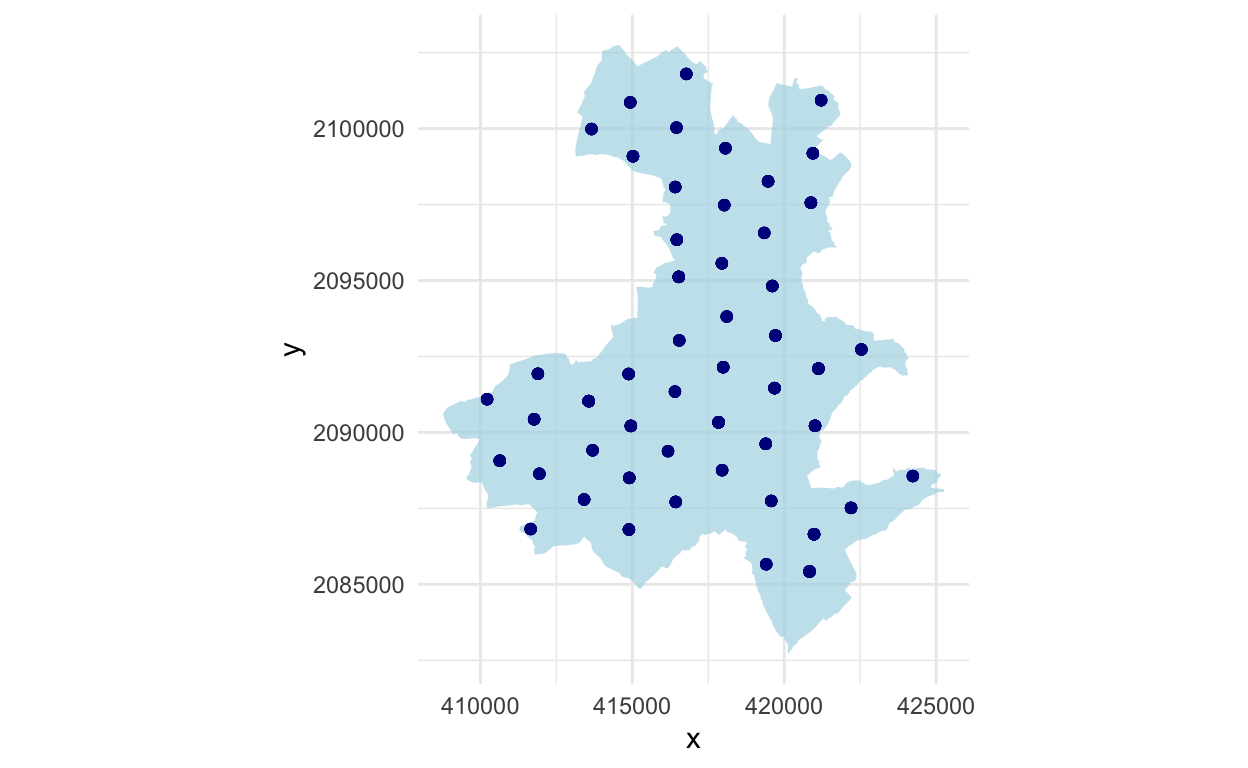Figure 1: Map with sampled points

Setting up the segment data, which in our case are the points that were visited…


# construct segment (point) data (x, y, Effort, Sample.Label)
segdata <- as.data.frame(matrix(NA, ncol = 5, nrow=100))
segdata <- DSdata[, c("Sample.Label", "Effort", "Point", "Xcoord", "Ycoord")]
segdata <- segdata[!duplicated(segdata), ]
colnames(segdata) <- c("Sample.Label", "Effort", "Segment.Label", "X", "Y")

Setting up the observation data, which links the observations with the segments (points):


obsdata <- DSdata
obsdata$size <- 1 obsdata$object <- 1:nrow(obsdata)
str(obsdata)

'data.frame':   575 obs. of  9 variables:
$Sample.Label: chr "Rouillacais_2015_1" "Rouillacais_2015_1" "Rouillacais_2015_1" "Rouillacais_2015_1" ...$ Point       : chr  "Rouillacais_2015_1" "Rouillacais_2015_1" "Rouillacais_2015_1" "Rouillacais_2015_1" ...
$Xcoord : int 419607 419607 419607 419607 419607 419341 419341 419341 417947 417947 ...$ Ycoord      : int  2094819 2094819 2094819 2094819 2094819 2096566 2096566 2096566 2095566 2095566 ...
$Area : num 1 1 1 1 1 1 1 1 1 1 ...$ distance    : num  NA 0.258 0.307 0.307 0.236 NA NA NA 0.131 0.158 ...
$Effort : num 3 3 3 3 3 3 3 3 3 3 ...$ size        : num  1 1 1 1 1 1 1 1 1 1 ...
$object : int 1 2 3 4 5 6 7 8 9 10 ... We then create the prediction grid (Figure 2).  # create a prediction grid # method from http://rfunctions.blogspot.co.uk/2014/12/how-to-create-grid-and-intersect-it.html library("raster") library("rgeos") library("dismo") # Create an empty raster grid <- raster(extent(shape)) # Choose its resolution. 500 m in both X and Y (truncation distance) res(grid) <- 500 # Make the grid have the same coordinate reference system (CRS) as the shapefile. proj4string(grid) <- proj4string(shape) # Transform this raster into a polygon and you will have a grid gridpolygon <- rasterToPolygons(grid) # Intersect our grid with shape pred.grid <- intersect(shape, gridpolygon) # Plot the intersected shape to check if everything is fine. plot(pred.grid)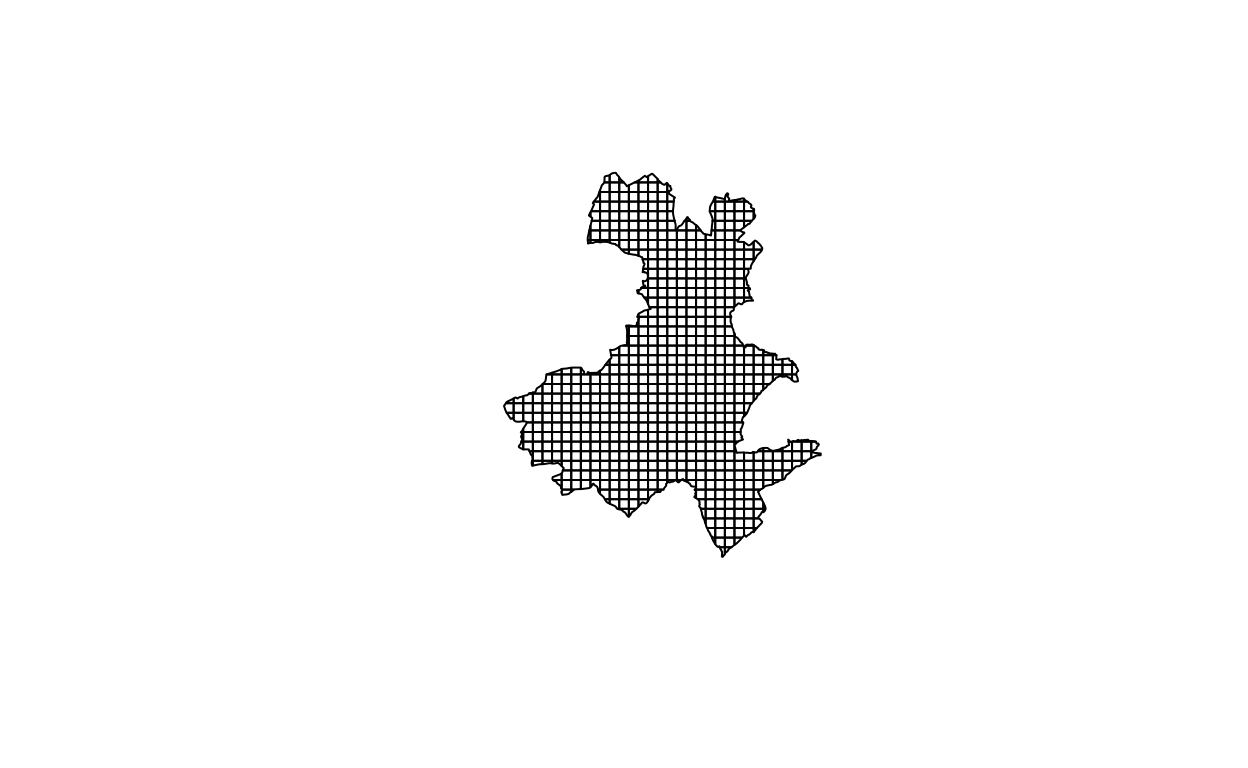Figure 2: Prediction grid  # create the data.frame for prediction preddata <- as.data.frame(matrix(NA, ncol=3, nrow=dim(pred.grid@data))) colnames(preddata) <- c("X", "Y", "area") for (i in 1:dim(pred.grid@data)){ preddata[i, c("X", "Y")] <- pred.grid@polygons[[i]]@labpt preddata[i, c("area")] <- pred.grid@polygons[[i]]@area/(1000^2) } The size of each cell in the prediction grid is 0.25 km$$^2$$ (created in metres and converted to kilometres). ## Detection function Detection functions can be fitted using the Distance R package:  library("Distance") # define distance bins cut <- c(0, 0.180, 0.220, 0.280, 0.300) df_ht <- ds(DSdata, truncation=0.3, transect="point", formula=~1, key="hn", adjustment=NULL, cutpoints=cut) We can look at a plot of the detection function and results from goodness of fit testing (Figure 3).  plot(df_ht, pdf=TRUE)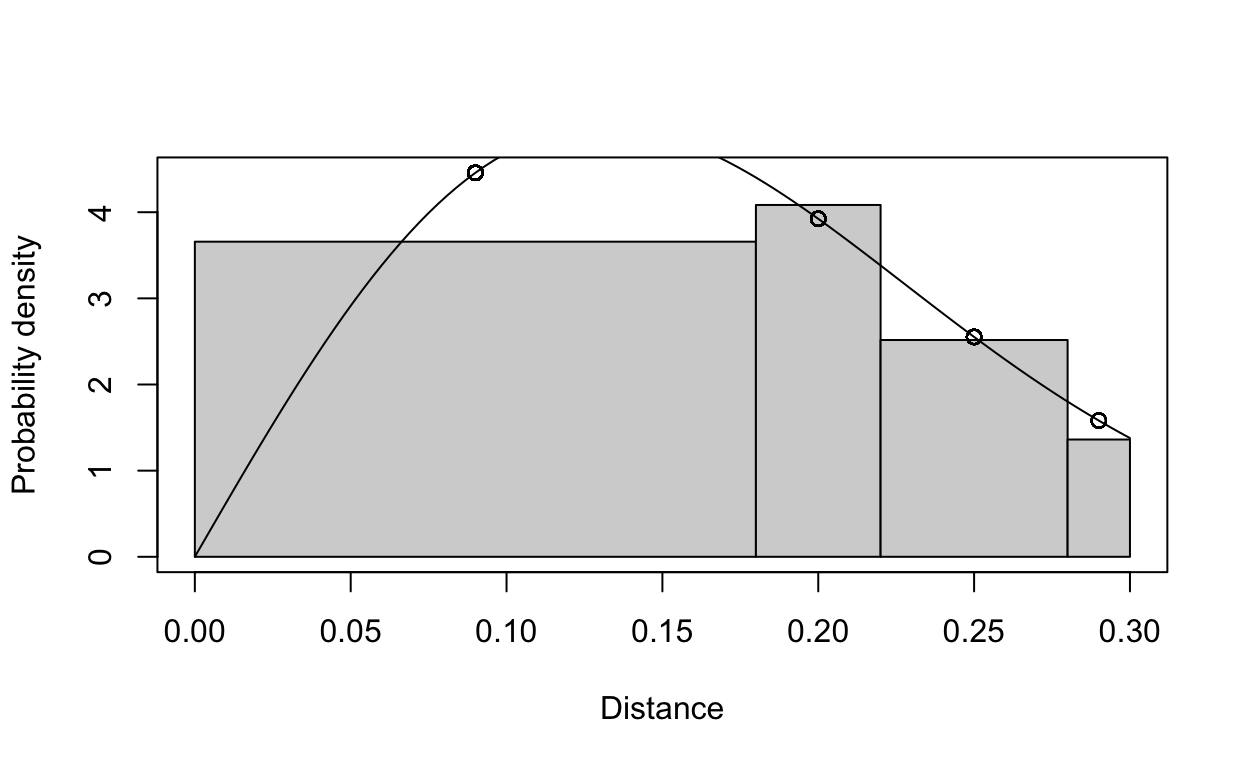Figure 3: Detection function: half-normal.  gof_ds(df_ht)  Goodness of fit results for ddf object Chi-square tests [0,0.18] (0.18,0.22] (0.22,0.28] (0.28,0.3] Observed 2.660000e+02 64.000000000 60.00000000 14.0000000 Expected 2.657738e+02 63.236416379 62.18979621 12.8000265 Chisquare 1.925852e-04 0.009220319 0.07710602 0.1124948 Total Observed 404.0000000 Expected 404.0000000 Chisquare 0.1990137 P = 0.90528 with 2 degrees of freedom We can see from the above, the model fit seems adequate. We can now fit some DSMs (Miller, Burt, Rexstad, & Thomas, 2013): ## Density surface modelling  library("dsm") Now fitting the DSMs, we model the count as a function of space, using a Tweedie (tw()) response distribution:  mod_tw <- dsm(count~s(X, Y), ddf.obj=df_ht, segment.data=segdata, observation.data=obsdata, family=tw(), transect="point") Note that we need to specify transect="point" to ensure that the effort is calculated properly. We can then look at the summary and model checking output (Figure 4).  summary(mod_tw)  Family: Tweedie(p=1.222) Link function: log Formula: count ~ s(X, Y) + offset(off.set) Parametric coefficients: Estimate Std. Error t value Pr(>|t|) (Intercept) 2.35638 0.09534 24.71 <2e-16 *** --- Signif. codes: 0 '***' 0.001 '**' 0.01 '*' 0.05 '.' 0.1 ' ' 1 Approximate significance of smooth terms: edf Ref.df F p-value s(X,Y) 20.61 25.28 2.996 6.12e-05 *** --- Signif. codes: 0 '***' 0.001 '**' 0.01 '*' 0.05 '.' 0.1 ' ' 1 R-sq.(adj) = 0.464 Deviance explained = 49.4% -REML = 254.02 Scale est. = 2.0851 n = 100  gam.check(mod_tw)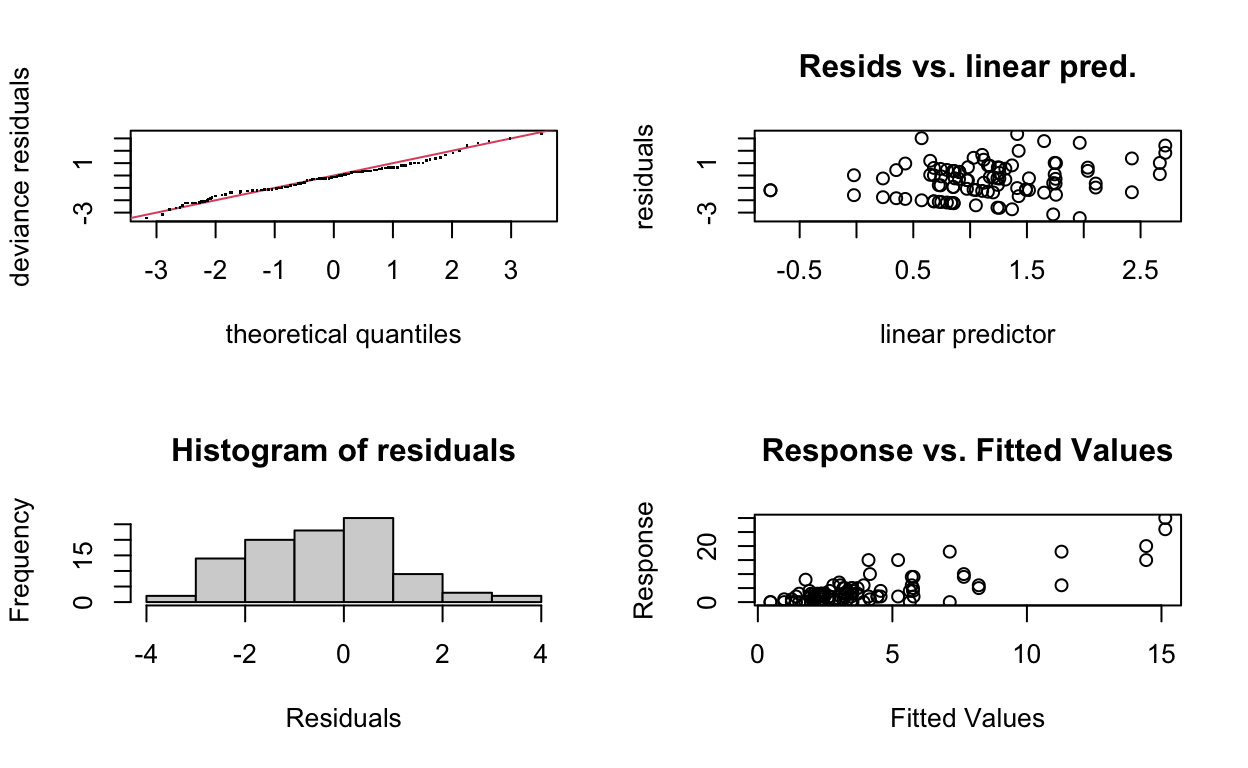Figure 4: Results from gam.check  Method: REML Optimizer: outer newton full convergence after 6 iterations. Gradient range [-3.695307e-08,7.842544e-08] (score 254.0232 & scale 2.085148). Hessian positive definite, eigenvalue range [3.066099,109.0183]. Model rank = 30 / 30 Basis dimension (k) checking results. Low p-value (k-index<1) may indicate that k is too low, especially if edf is close to k'. k' edf k-index p-value s(X,Y) 29.0 20.6 1.13 0.99 This model is fairly rudimentary, so the plot of response vs fitted values doesn’t seem that great (note the difference in axis scales) but for illustration purposes here we accept what we have. ## Making predictions We can now predict over the prediction grid.  mod_tw_pred <- predict(mod_tw, preddata, preddata$area)

Here we define a convenience function to generate an appropriate data structure for ggplot2 to plot: given the argument fill (the covariate vector to use as the fill) and a name, return a geom_polygon object (fill must be in the same order as the polygon data).


grid_plot_obj <- function(shape,fill, name){

# what data were supplied?
names(fill) <- NULL
row.names(fill) <- NULL
data <- data.frame(fill)
names(data) <- name

# ! need to give the right name of the shapefile
sp <- shape
spdf <- SpatialPolygonsDataFrame(sp, data)
spdf@data$id <- rownames(spdf@data) spdf.points <- fortify(spdf, region="id") spdf.df <- join(spdf.points, spdf@data, by="id") # store the x/y even when projected and labelled as "long" and "lat" spdf.df$x <- spdf.df$long spdf.df$y <- spdf.df$lat geom_polygon(aes_string(x="x",y="y",fill=name, group="group"), data=spdf.df) } # make the plot pcount_tw <- ggplot() + grid_plot_obj(pred.grid, mod_tw_pred, "Density") + scale_fill_gradient(low="white", high="chocolate4") + coord_equal() + theme_minimal() + geom_path(aes(x=x, y=y), data=survey.area) + geom_point(aes(x = Xcoord, y = Ycoord, group="Point"), data = DSdata, colour = "black") + labs(fill="Density") We can also estimate uncertainty in our abundance map in the form of a map of coefficients of variation. Note that since there are no covariates in the detection function, we use the dsm.var.gam function to estimate the variance (if there were covariates varying at the segment level, such as sea state or observer, we could use dsm.var.prop) (Figure 5).  # data setup for plotting preddata.var <- split(preddata, 1:nrow(preddata)) # estimate variance mod_tw_var <- dsm.var.gam(mod_tw, pred.data=preddata.var, off.set=preddata$area)
summary(mod_tw_var)

Summary of uncertainty in a density surface model calculated
analytically for GAM, with delta method

Approximate asymptotic confidence interval:
2.5%     Mean    97.5%
1588.720 2010.275 2543.686
(Using log-Normal approximation)

Point estimate                 : 2010.275
CV of detection function       : 0.06566247
CV from GAM                    : 0.101
Total standard error           : 242.2565
Total coefficient of variation : 0.1205 

# plot
pcount_cv_tw <- ggplot() +
grid_plot_obj(pred.grid, sqrt(mod_tw_var$pred.var)/unlist(mod_tw_var$pred), "CV") +
scale_fill_gradient(low = "white", high = "chocolate4") +
coord_equal() + theme_minimal() +
geom_path(aes(x=x, y=y),data=survey.area) +
geom_point(aes(x=Xcoord, y=Ycoord, group="Point"), data=DSdata, colour="black")

library("gridExtra")
grid.arrange(pcount_tw, pcount_cv_tw, ncol=2)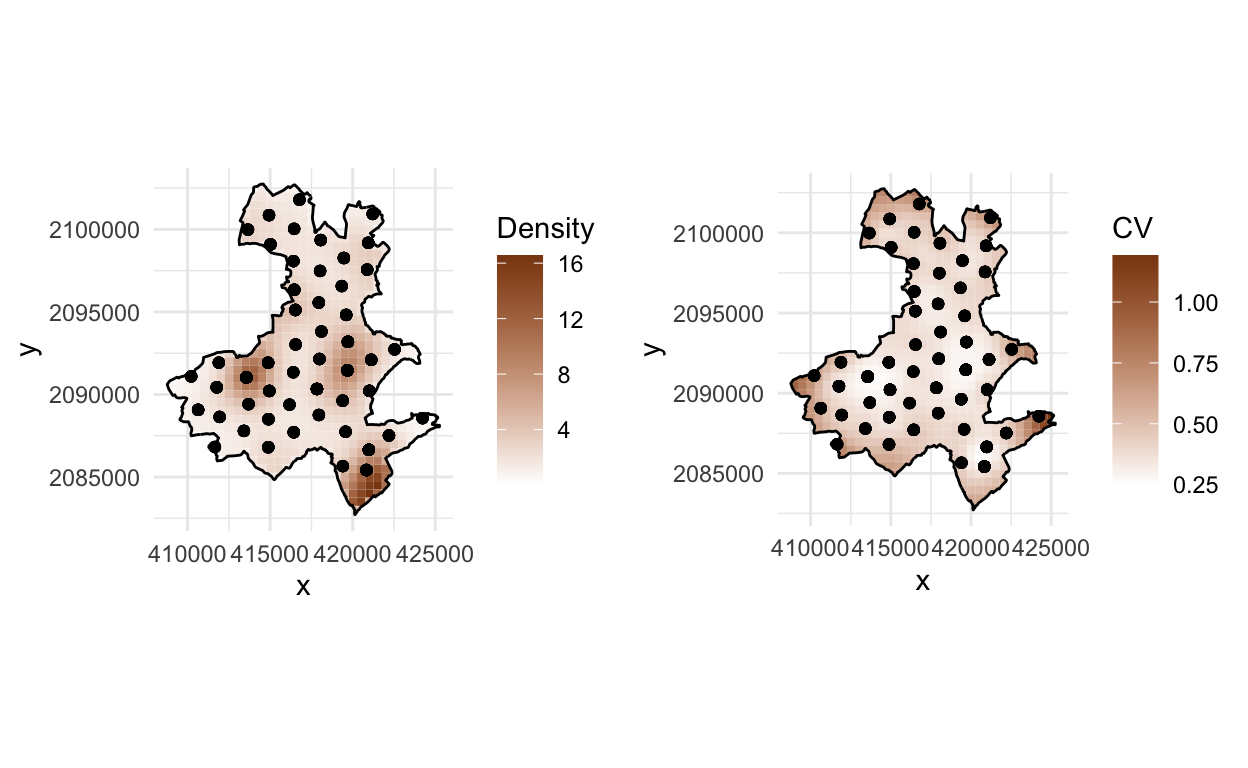Figure 5: Map of hare density (ind/km²) and of associated coefficient of variation in Rouillacais site from the DSM model with coordinates as covariates using Tweedie response. Black dots represent the sampled point.

This is a small example of what can be done using DSM and the simplest covariates available: geographical coordinates.

Mauvy, B., Souchay, G., Arnauduc, J.-P., & Guitton, J.-S. (2017). The french hare network : A tool to study european hare populations dynamics on a large scale. 33rd iubg congress & 14th perdix symposium. Retrieved from http://iugb2017.com/wp-content/uploads/2017/08/abstract-book-FINAL-VERSION.pdf

Miller, D. L., Burt, M. L., Rexstad, E. A., & Thomas, L. (2013). Spatial models for distance sampling data: Recent developments and future directions. Methods in Ecology and Evolution, 4, 1001–1010. https://doi.org/10.1111/2041-210X.12105

Peroux, R., Mauvy, B., Lartiges, A., Bray, Y., & Marboutin, E. (1997). Point transects sampling: A new approach to estimate densities or abundances of european hare (lepus europaeus) from spotlight counts. Game and Wildlife, 14, 525–529.

R Core Team. (2019). R: A language and environment for statistical computing. Vienna Austria: R Foundation for Statistical Computing.